Application

# What are the disadvantages and remedies of IMU?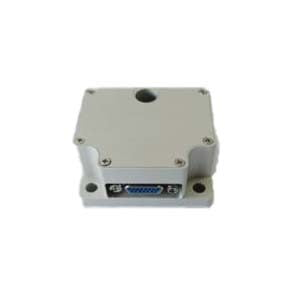IMU (English Inertial measurement unit) is a device used to measure object’s three-axis attitude Angle and acceleration. Common IMUs include a three-axis gyroscope and a three-axis accelerometer, and some 9-axis IMUs also include a three-axis magnetometer.

Working principle of accelerometer:

1.Model equivalence: the accelerometer can be represented by a simple mass block + spring + indicator, as shown in the figure below.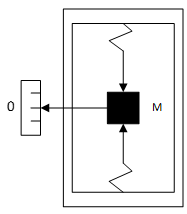Measurement model of accelerometer

The measured value of acceleration is am, which is the acceleration corresponding to the tension of the spring: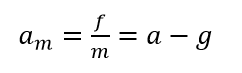f the pull of the spring, the acceleration of the object a in inertial coordinates, and g the acceleration of gravity (where g is a vector).

2.Measurement principle of accelerometer

Usually, we assume that the earth surface is the inertial reference frame, but for high-end measurement units, such accuracy is far from enough (the effect of earth rotation needs to be taken into account), so the origin of the inertial reference frame is denoted as the earth’s center of mass, and the coordinate system located on the Earth surface is called the ground reference frame or the northeast sky coordinate system (ENU coordinate system), as shown in the figure below.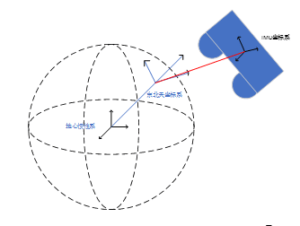Working principle of gyroscope

1.Model equivalence

In the rotating coordinate system, the moving object is subjected to Coriolis force (refer to motion rotatology). Taking MEMS gyroscope as an example, it is mainly divided into: an active axis of motion + a sensitive axis.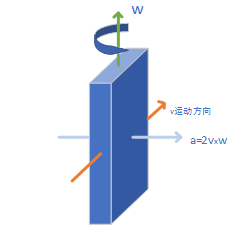2.The gyroscope model

In the general process, tuning fork gyroscope is generally used, because the object itself may have a certain acceleration in the direction of Coriolis force, so the tuning fork gyroscope is used to form a difference model and eliminate its own influence.

IMU classification

Imus can be divided into RLG IMUs,FOG IMUs and MEMS IMUs according to the different core sensors used.

The main disadvantage of using IMU navigation is that they usually accumulate errors, including Abbe errors. Because the guidance system will continue to add the position results calculated by the detection to the original position information (see trajectory calculation), although the errors in the measurement are small, these errors continue from one position to another. Accumulation leads to “drift”, or the difference between the system’s perceived location and the actual location becomes larger and larger. Location tracking systems (such as the Global Positioning System (GPS)) can be used to continuously correct offset errors.

Because the device can only collect data in a limited time interval, IMU always uses average values in its work. If an accelerometer can retrieve acceleration at a frequency of once per second, the device will assume that the acceleration is always a value in this second, although the acceleration may vary greatly during this period. Due to integration, the constant error will increase at a quadratic linear rate in the acceleration result.

Through the inertial measurement unit, we can obtain the posture and angular velocity of the object in three-dimensional space. Using other equipment combined with IMU (such as Global Positioning System GPS), we can also extend the application of IMU to more fields. I believe that with the development of technology, inertial measurement units can be better used in our lives in the future.